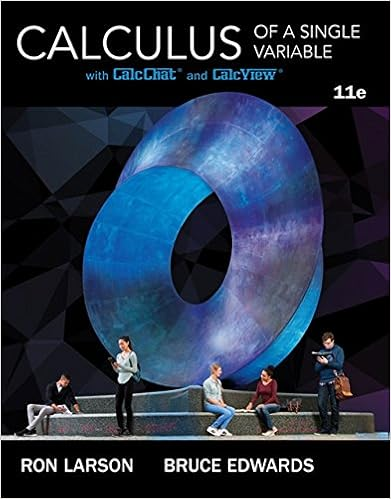# Evaluate z c f ds where f x y z y2 x z2 and c is the

• Test Prep
• 3
• 80% (5) 4 out of 5 people found this document helpful

This preview shows page 1 - 3 out of 3 pages.

##### We have textbook solutions for you!
The document you are viewing contains questions related to this textbook.The document you are viewing contains questions related to this textbook.
Chapter 9 / Exercise 71
Calculus of a Single Variable
Edwards/LarsonExpert Verified
5. Evaluate~c
6. LetSbe the portion of the paraboloidz= 4-x2-y2for whichz0 with upwardorientation and let~cbe the positively oriented circlex2+y2= 4 that forms theboundary ofSin thexy-plane.Use Stokes’ Theorem to compute the path integralZ~c~F·d~s, where~F(x, y, z) = (2z,3x,5y).
##### We have textbook solutions for you!
The document you are viewing contains questions related to this textbook.The document you are viewing contains questions related to this textbook.
Chapter 9 / Exercise 71
Calculus of a Single Variable
Edwards/LarsonExpert Verified
7. Determine whether the given sequence is convergent or divergent. If convergent, findthe limit. If divergent, explain why?
•••